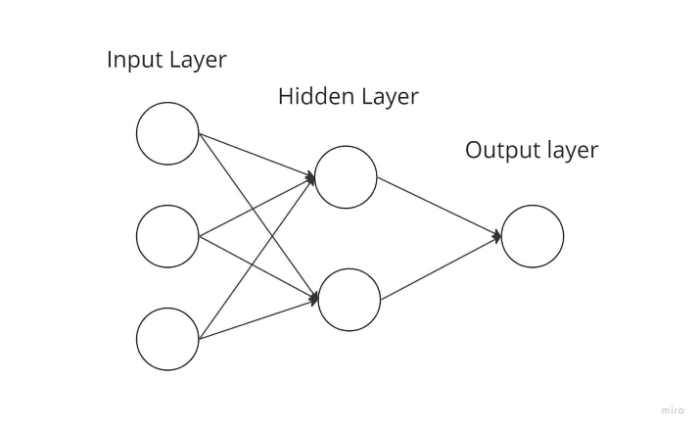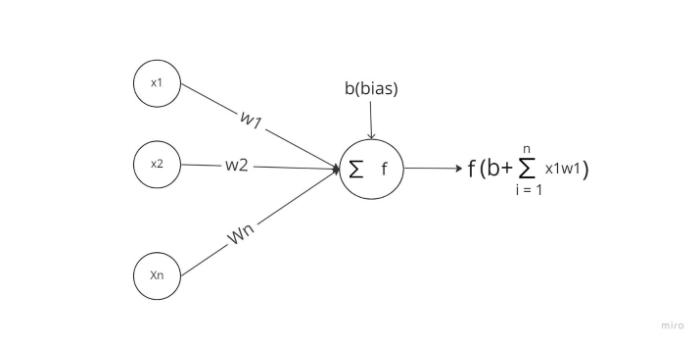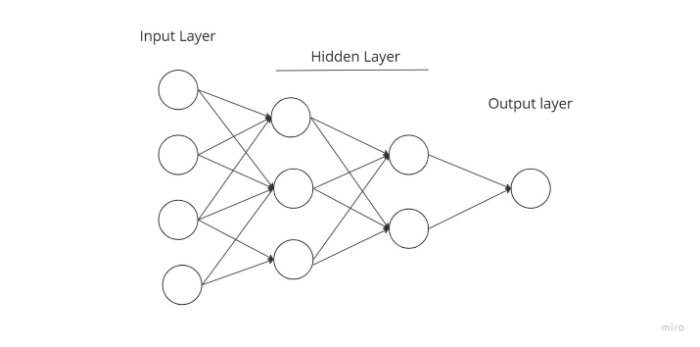# Understanding Multi-Layer Feed-Forward Neural Networks in Machine Learning

Deep-learning feed-forward neural networks are used in a variety of applications, including computer assistants, search engines, and machine translation. They serve as the foundation for several significant neural networks used today, including recurrent neural networks, which are widely used in natural language processing and sequence learning, and convolutional neural networks, which are widely used in computer vision applications. In this article, we'll discuss multi-layer feed-forward neural networks.

## What is Feed Forward Neural Network?

The most fundamental kind of neural network, in which input data travels only in one way before leaving through output nodes and passing through artificial neural nodes. Input and output layers are present in locations where hidden layers may or may not be present. Based on this, they are further divided into single-layered and multi-layered feed-forward neural networks.The complexity of the function is inversely correlated with the number of layers. It cannot spread backward; it can only go forward. In this scenario, the weights are unchanged. Weights are added to the inputs before being passed to an activation function.

## Feed Forward Neural Network components

### Neurons

The fundamental component of a neural network is an artificial neuron. The following is a schematic illustration of a neuron.It operates in two parts, as can be seen above: first, it computes the weighted sum of its inputs, and then it uses an activation function to normalize the total. There are both linear and nonlinear activation functions. Additionally, each input to a neuron has a corresponding weight. The network must learn these parameters during the training phase.

### Activation Function

A neuron's activation function serves as a decision-making body at the output. The activation function determines whether the neuron learns linear or non-linear decision limits. Additionally, it has a leveling impact on neuron output, preventing the cascade effect from making neurons' output after multiple layers become exceedingly enormous. The three most popular activation mechanisms are as follows −

• Sigmoid − Mapped to the output values are input values between 0 and 1.
• Tanh − The input values are mapped to a value in the range of -1 and 1.
• ReLu − This function only allows positive numbers to pass through it. Inverse values are assigned to 0.

### Input layer

This layer's neurons take in information and send it to the network's other levels. The number of neurons in the input layer must equal the number of features or attributes in the dataset.

### Output Layer

This layer is the one that provides the predictions. For various issues, a distinct activation function should be used in this layer. We want the result to be either 0 or 1 for a binary classification task. As a result, the sigmoid activation function is employed. A Softmax (think of it as a sigmoid applied to several classes) is used for multiclass classification problems. We can utilize a linear unit to solve regression problems where the result does not fall into a predetermined category.

### Hidden layer

Layers concealed between the input and output are used to segregate them. The number of hidden layers will depend on the type of model. In order to actually transfer the input to the next layer, numerous neurons in hidden layers first modify it. For the purpose of improving predictability, this network's weights are adjusted continuously.

## Working principle of Feed Forward Neural Network

A single-layer perceptron could represent how the feed-forward neural network looks when it is simplified. As inputs enter the layer, this model multiplies them with weights. The total is then obtained by adding the weighted input values collectively. In general, the output value is 1, and if the total of the values falls below the threshold, it is typically -1, as long as the sum is above a predetermined threshold, which is set at zero.

The single-layer perceptron is a feed-forward neural network model that is frequently used for classification. Single-layer perceptrons can also benefit from machine learning. Neural networks can modify their weights during training based on a characteristic known as the delta rule, which allows them to compare their outputs to the expected values. Gradient descent is the consequence of training and learning. Multi-layered perceptrons update their weights in the same way. However, this is known as back-propagation. In this situation, the network's hidden layers will be changed based on the final layer's output values.

## Multi-layer Feed Forward Neural Network

An entrance point into sophisticated neural networks, where incoming data is routed through several layers of artificial neurons. Every node is linked to every neuron in the following layer, resulting in a fully connected neural network. There are input and output layers, as well as several hidden levels, for a total of at least three or more layers. It possesses bidirectional propagation, which means it can propagate both forward and backward.Inputs are multiplied by weights and supplied into the activation function, where they are adjusted to minimize loss during backpropagation. Weights are just machine-learned values from Neural Networks. They modify themselves based on the gap between projected and training outcomes. Softmax is used as an output layer activation function after nonlinear activation functions.

## Conclusion

Finally, feedforward neural networks are sometimes referred to as Multi-layered Networks of Neurons (MLN). The information only flows forward in the neural network, first through the input nodes, then through the hidden layers (single or many layers), and ultimately through the output nodes, which is why this network of models is termed feedforward.

Updated on: 28-Oct-2022

5K+ Views